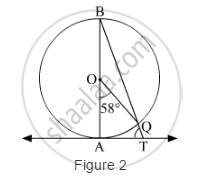# In Fig. 2, AB is the diameter of a circle with centre O and AT is a tangent. If ∠AOQ = 58°, find ∠ATQ. - Mathematics

In Fig. 2, AB is the diameter of a circle with centre O and AT is a tangent. If ∠AOQ = 58°, find ∠ATQ.#### Solution

We know that the angle subtended by an arc of a circle at the centre is twice the angle subtended by it at any point on the remaining part of the circle.

AOQ = 2ABQ

ABQ =1/2AOQ

ABQ =1/2×58°=29°

or ABT = 29°

We know that the radius is perpendicular to the tangent at the point of contact.

OAT = 90°     (OA ⊥ AT)

or BAT = 90°

Now, in ∆BAT,

∠BAT+∠ABT+∠ATB=180°

⇒90°+29°+∠ATB=180°

⇒∠ATB=180°−119°=61°

∴∠ATQ=61°

Concept: Concept of Circle - Centre, Radius, Diameter, Arc, Sector, Chord, Segment, Semicircle, Circumference, Interior and Exterior, Concentric Circles
Is there an error in this question or solution?